# Thin Rolled Section Civil Engineering (CE) Notes | EduRev

## Civil Engineering (CE) : Thin Rolled Section Civil Engineering (CE) Notes | EduRev

The document Thin Rolled Section Civil Engineering (CE) Notes | EduRev is a part of the Civil Engineering (CE) Course Advanced Solid Mechanics - Notes, Videos, MCQs & PPTs.
All you need of Civil Engineering (CE) at this link: Civil Engineering (CE)

Thin rolled section

Here we study the problem of end torsion of a bar whose cross section is narrow and has small curvature such as those shown in figure 9.20. Such sections are usually made of hot rolled steel and hence are called rolled sections. Since, the shape across the thickness is similar to that of the thin rectangular section, excepting at the bent corners, it is assumed that the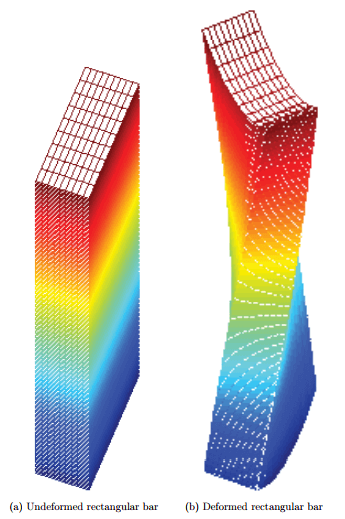Figure 9.18: A bar with rectangular cross section subjected to end twisting moment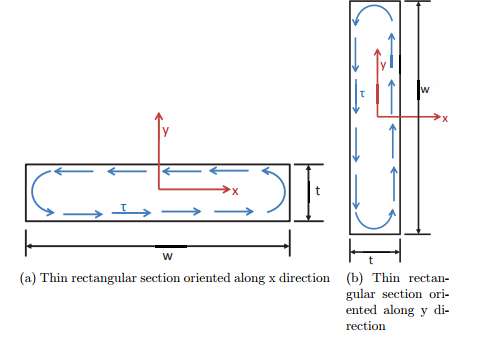Figure 9.19: Schematic of shear stress distribution in a thin rectangular section in different orientations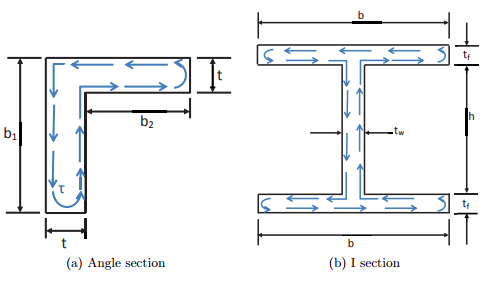Figure 9.20: Schematic of shear stress distribution in a thin rolled sections

solution obtained for thin rectangular section holds. In order for the corners not to change the displacement and stress field from that obtained for a thin rectangular section, it is required that the radius of curvature of these corners be large.

Thus, for the angle section shown in figure 9.20a, the torque required to realize a given angle of twist per unit length and the maximum shear stress in the section is obtained from (9.95) as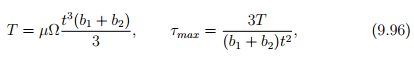where we have treated the entire angle without the 90 degree bend, as a thin walled rectangular section. In order for the above equations to be reasonable, (b1 + b2)/t > 10 and the radius of the curvature of the bent corner large.

Similarly, for the I section shown in figure 9.20b, the torque required to realize a given angle of twist per unit length and the maximum shear stress in the section is obtained from (9.95) as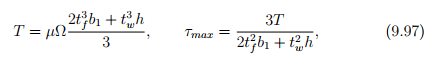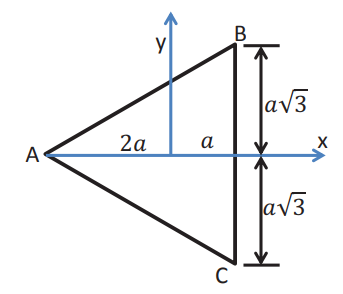Figure 9.21: Orientation of an equilateral triangle with respect to a given coordinate basis

where the torsional stiffness due to each of the three plates - the two flange plates and the web plate - is summed algebraically. This is justified because the shear stress distribution in each of these plates is similar to that of a thin rectangular section subjected to torsion, as indicated in the figure 9.20b. However, comparison of this solution with the exact result for this geometry is beyond the scope of this notes.

Offer running on EduRev: Apply code STAYHOME200 to get INR 200 off on our premium plan EduRev Infinity!

## Advanced Solid Mechanics - Notes, Videos, MCQs & PPTs

42 videos|61 docs

,

,

,

,

,

,

,

,

,

,

,

,

,

,

,

,

,

,

,

,

,

;